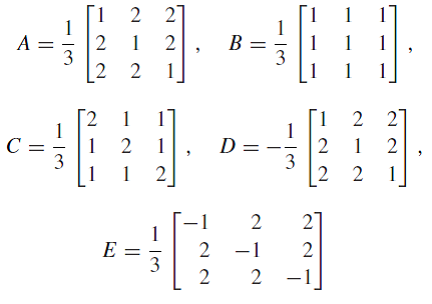Ask question

# Justify Conclusions take a conjecture about the sum of th interior angles of a quadrilateral. Justify your reasoning.# Justify Conclusions take a conjecture about the sum of th interior angles of a quadrilateral. Justify your reasoning.

Question
Congruenceasked 2021-03-05
Justify Conclusions take a conjecture about the sum of th interior angles of a quadrilateral. Justify your reasoning.

## Answers (1)2021-03-06
A quadrilateral can be divided into two triangles but drawing one of its diagonals. Since the sum of the interior angles of a triangle is 180°, then we conjecture that the sum of the interior angles of a quadrilateral is $$\displaystyle{2}{\left({180}°\right)}={360}°.$$

### Relevant Questionsasked 2021-03-26

One of the five given matrices represents an orthogonal projection onto a line and another represents a reflection about line. Identify both and briefly justify your choice.asked 2021-03-15
Find a complete set of mutually incogruent solutions.
$$\displaystyle{9}{x}\equiv{12}\pm{o}{d}{\left\lbrace{15}\right\rbrace}$$asked 2021-03-23
Find a complete set of mutually incogruent solutions.
$$\displaystyle{7}{x}\equiv{5}\pm{o}{d}{\left\lbrace{11}\right\rbrace}$$asked 2021-02-27
A Counterexample to the statement, If $$\displaystyle{a}\equiv{b}\pm{o}{d}{m}$$ and $$\displaystyle{c}\equiv{d}\pm{o}{d}{m}$$, where a,b,c,d, and m are integers with c and d positive and $$\displaystyle{m}\geq{2}$$, then $$\displaystyle{a}^{{{c}}}\equiv{b}^{{{d}}}\pm{o}{d}{m}$$.asked 2021-04-30
A Counterexample to the statement, If $$\displaystyle{a}{c}\equiv{b}{c}\pm{o}{d}{m}$$, where a,b,c, and m are integers with $$\displaystyle{m}\geq{2}$$, then $$\displaystyle{a}\equiv{b}\pm{o}{d}{m}$$.asked 2021-05-06
To determine: The smallest nonnegative integer x that satisfies the given system of congruences.
$$\displaystyle{x}\equiv{1}\pm{o}{d}{\left\lbrace{4}\right\rbrace}$$
$$\displaystyle{x}\equiv{8}\pm{o}{d}{\left\lbrace{9}\right\rbrace}$$
$$\displaystyle{x}\equiv{10}\pm{o}{d}{\left\lbrace{25}\right\rbrace}$$asked 2021-03-25
To determine: The smallest nonnegative integer x that satisfies the given system of congruences.
$$\displaystyle{x}\equiv{1003}\pm{o}{d}{\left\lbrace{17},{369}\right\rbrace}$$
$$\displaystyle{x}\equiv{2974}\pm{o}{d}{\left\lbrace{5472}\right\rbrace}$$asked 2021-05-04
To determine: The smallest nonnegative integer x that satisfies the given system of congruences.
$$\displaystyle{x}\equiv{3}\pm{o}{d}{\left\lbrace{1917}\right\rbrace}$$
$$\displaystyle{x}\equiv{75}\pm{o}{d}{\left\lbrace{385}\right\rbrace}$$asked 2021-04-19
To determine: The smallest nonnegative integer x that satisfies the given system of congruences.
$$\displaystyle{x}\equiv{6}\pm{o}{d}{8}$$
$$\displaystyle{x}\equiv{17}\pm{o}{d}{\left\lbrace{25}\right\rbrace}$$asked 2021-04-16
To determine: The smallest nonnegative integer x that satisfies the given system of congruences.
$$\displaystyle{x}\equiv{3}\pm{o}{d}{5}$$
$$\displaystyle{x}\equiv{7}\pm{o}{d}{8}$$
...